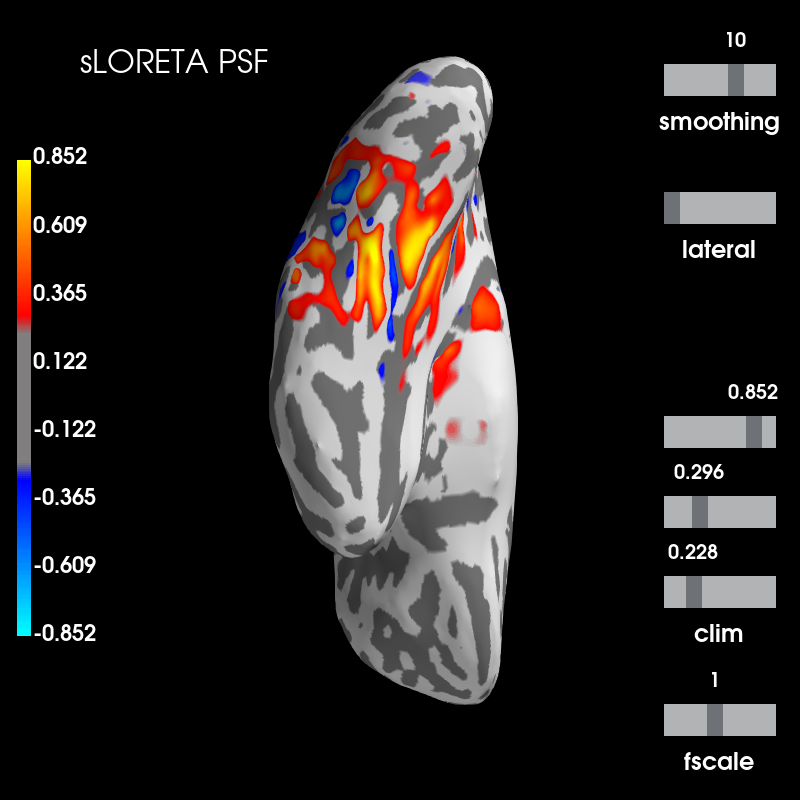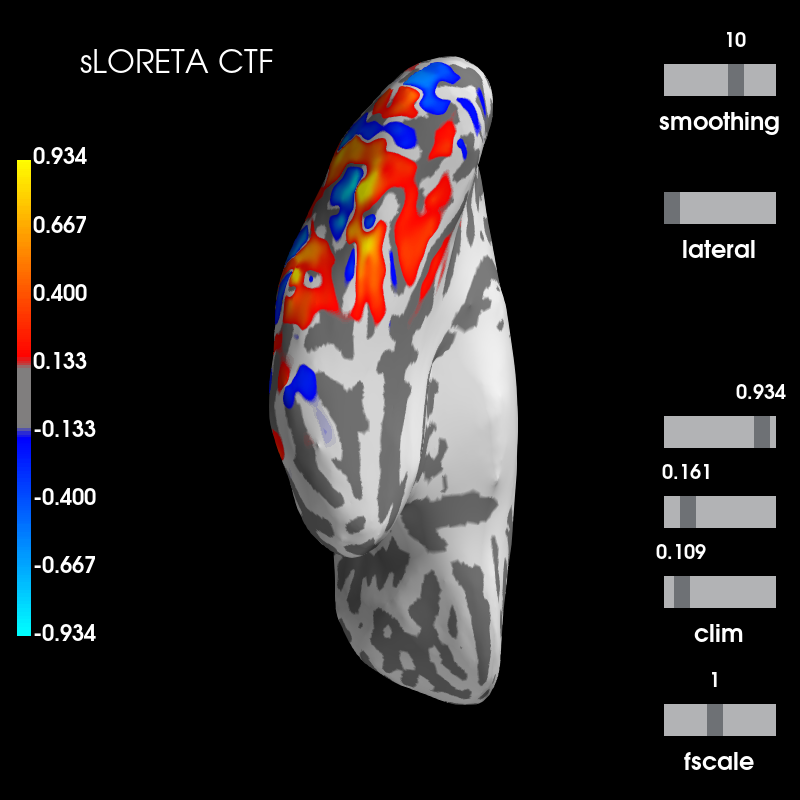# Plot point-spread functions (PSFs) and cross-talk functions (CTFs)¶

Visualise PSF and CTF at one vertex for sLORETA.

# Authors: Olaf Hauk <olaf.hauk@mrc-cbu.cam.ac.uk>
#          Alexandre Gramfort <alexandre.gramfort@inria.fr>
#

import mne
from mne.datasets import sample
from mne.minimum_norm import (make_inverse_resolution_matrix, get_cross_talk,

print(__doc__)

data_path = sample.data_path()
subjects_dir = data_path + '/subjects/'
fname_fwd = data_path + '/MEG/sample/sample_audvis-meg-eeg-oct-6-fwd.fif'
fname_cov = data_path + '/MEG/sample/sample_audvis-cov.fif'
fname_evo = data_path + '/MEG/sample/sample_audvis-ave.fif'

# forward operator with fixed source orientations
forward = mne.convert_forward_solution(forward, surf_ori=True,
force_fixed=True)

# noise covariance matrix

# evoked data for info

# make inverse operator from forward solution
# free source orientation
inverse_operator = mne.minimum_norm.make_inverse_operator(
info=evoked.info, forward=forward, noise_cov=noise_cov, loose=0.,
depth=None)

# regularisation parameter
snr = 3.0
lambda2 = 1.0 / snr ** 2
method = 'MNE'  # can be 'MNE' or 'sLORETA'

# compute resolution matrix for sLORETA
rm_lor = make_inverse_resolution_matrix(forward, inverse_operator,
method='sLORETA', lambda2=lambda2)

# get PSF and CTF for sLORETA at one vertex
sources = 

stc_psf = get_point_spread(rm_lor, forward['src'], sources, norm=True)

stc_ctf = get_cross_talk(rm_lor, forward['src'], sources, norm=True)


Out:

Reading forward solution from /home/circleci/mne_data/MNE-sample-data/MEG/sample/sample_audvis-meg-eeg-oct-6-fwd.fif...
Computing patch statistics...
[done]
Computing patch statistics...
[done]
Desired named matrix (kind = 3523) not available
Read MEG forward solution (7498 sources, 306 channels, free orientations)
Desired named matrix (kind = 3523) not available
Read EEG forward solution (7498 sources, 60 channels, free orientations)
MEG and EEG forward solutions combined
Source spaces transformed to the forward solution coordinate frame
Average patch normals will be employed in the rotation to the local surface coordinates....
Converting to surface-based source orientations...
[done]
366 x 366 full covariance (kind = 1) found.
Read a total of 4 projection items:
PCA-v1 (1 x 102) active
PCA-v2 (1 x 102) active
PCA-v3 (1 x 102) active
Average EEG reference (1 x 60) active
Read a total of 4 projection items:
PCA-v1 (1 x 102) active
PCA-v2 (1 x 102) active
PCA-v3 (1 x 102) active
Average EEG reference (1 x 60) active
Found the data of interest:
t =    -199.80 ...     499.49 ms (Left Auditory)
0 CTF compensation matrices available
nave = 55 - aspect type = 100
Projections have already been applied. Setting proj attribute to True.
No baseline correction applied
Computing inverse operator with 364 channels.
364 out of 366 channels remain after picking
Selected 364 channels
Whitening the forward solution.
Created an SSP operator (subspace dimension = 4)
Computing rank from covariance with rank=None
Using tolerance 3.3e-13 (2.2e-16 eps * 305 dim * 4.8  max singular value)
Estimated rank (mag + grad): 302
MEG: rank 302 computed from 305 data channels with 3 projectors
Using tolerance 4.7e-14 (2.2e-16 eps * 59 dim * 3.6  max singular value)
Estimated rank (eeg): 58
EEG: rank 58 computed from 59 data channels with 1 projector
Setting small MEG eigenvalues to zero (without PCA)
Setting small EEG eigenvalues to zero (without PCA)
Creating the source covariance matrix
Computing SVD of whitened and weighted lead field matrix.
largest singular value = 4.31738
scaling factor to adjust the trace = 1.68644e+20
364 out of 366 channels remain after picking
Preparing the inverse operator for use...
Scaled noise and source covariance from nave = 1 to nave = 1
Created the regularized inverter
Created an SSP operator (subspace dimension = 4)
Created the whitener using a noise covariance matrix with rank 360 (4 small eigenvalues omitted)
Computing noise-normalization factors (sLORETA)...
[done]
Applying inverse operator to ""...
Picked 364 channels from the data
Computing inverse...
Eigenleads need to be weighted ...
Computing residual...
Explained  43.2% variance
sLORETA...
[done]
Dimension of Inverse Matrix: (7498, 364)
Dimensions of resolution matrix: 7498 by 7498.


## Visualise¶

# Which vertex corresponds to selected source
vertno_lh = forward['src']['vertno']
verttrue = [vertno_lh[sources]]  # just one vertex

# find vertices with maxima in PSF and CTF
vert_max_psf = vertno_lh[stc_psf.data.argmax()]
vert_max_ctf = vertno_lh[stc_ctf.data.argmax()]

brain_psf = stc_psf.plot('sample', 'inflated', 'lh', subjects_dir=subjects_dir,
figure=1)
brain_psf.show_view('ventral')
brain_psf.add_text(0.1, 0.9, 'sLORETA PSF', 'title', font_size=16)

# True source location for PSF
color='green')

# Maximum of PSF
hemi='lh', color='black')

brain_ctf = stc_ctf.plot('sample', 'inflated', 'lh', subjects_dir=subjects_dir,
figure=2)
brain_ctf.add_text(0.1, 0.9, 'sLORETA CTF', 'title', font_size=16)
brain_ctf.show_view('ventral')
color='green')

# Maximum of CTF
hemi='lh', color='black')

print('The green spheres indicate the true source location, and the black '
'spheres the maximum of the distribution.')Out:

Using control points [0.22842778 0.2956258  0.85246681]
Using control points [0.10932369 0.16149934 0.93351158]
The green spheres indicate the true source location, and the black spheres the maximum of the distribution.


Total running time of the script: ( 0 minutes 8.952 seconds)

Estimated memory usage: 448 MB

Gallery generated by Sphinx-Gallery